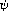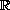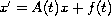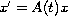Electron. J. Diff. Eqns., Vol. 2007(2007), No. 52, pp. 1-10.

### Existence of-bounded solutions for nonhomogeneous linear differential equations Pham Ngoc Boi

Abstract:
In this article we present a necessary and sufficient condition for the existence of-bounded solution onof the nonhomogeneous linear differential equation. We associate that with the condition of the concept-dichotomy onof the homogeneous linear differential equation.

Submitted January 26, 2007. Published April 5, 2007.
Math Subject Classifications: 34A12, 34C11, 34D05.
Key Words: \psi-bounded solution; \psi-integrable; \psi-integrally bounded; \psi-exponential dichotomy.

Show me the PDF file (227K), TEX file, and other files for this article.Pham Ngoc Boi Department of Mathematics Vinh University Vinh City, Vietnam email: pnboi_vn@yahoo.com